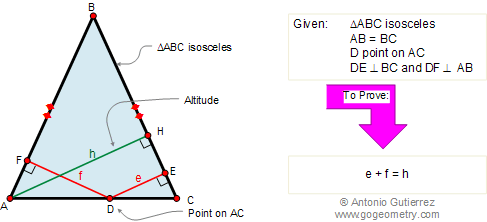Problem 223. Viviani's Theorem, Isosceles Triangle, Altitude, Distance
 In an isosceles triangle ABC (AB = BC), the sum of the distances from any point on AC to the equal sides is equal to the altitude of the equal sides. View or post a solution.The theorem is named for Vincenzo Viviani (1622-1703), a pupil of Galileo and Torricelli, who is also remembered for a reconstruction of a book on the conic sections of Apollonius and for finding a way of trisecting an angle through the use an equilateral hyperbola.

 Home | Geometry | Search | Problems | 221-230 | Area of a Triangle | Email | View or post a solution